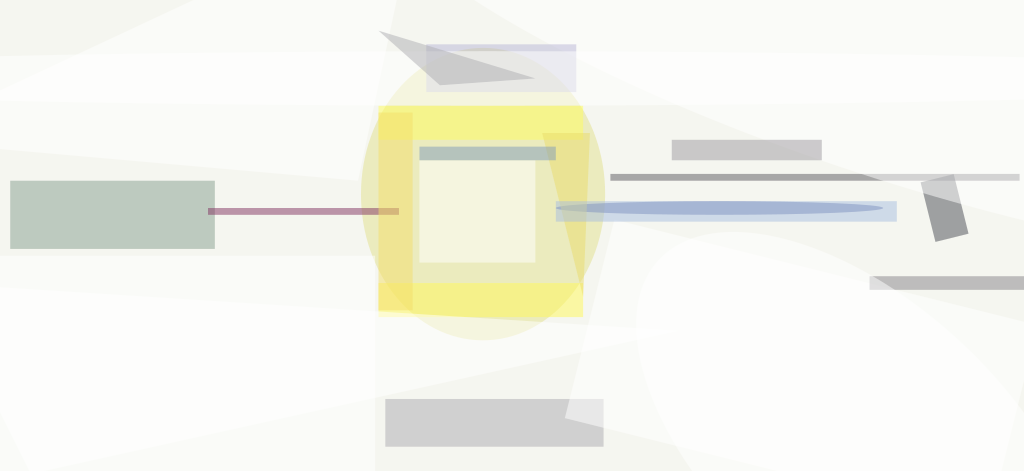Aset of equations has been formulated to enable quantification of several aspects of the performance of an analog photonic communication link as affected by laser noise. A procedure for measuring the laser noise has been devised to provide laser-noise data for use in the equations. The equations and procedure are generally applicable to diverse analog photonic links, in the design and operation of which laser noise is of great concern. Analog photonic links have been found to be useful as subsystems, radio-antenna-arraying systems, optoelectronic oscillators, and wide-band signal-processors.Figure 1. In an Analog Photonic Link of the type of interest here, RF intensity modulation is effected by use of a Mach-Zehnder modulator and the RF signal is detected by a photodiode. Figure 1 schematically depicts a link of the type to which the equations apply. At the transmitter, the continuous-wave output of a laser is intensity-modulated at a radio frequency (RF) by means of a Mach-Zehnder modulator. The resulting modulated laser beam is conveyed via an optical fiber to the receiver, where the RF signal is detected directly by use of a photodiode.

The equations are derived from first principles, using what is known of (1) the optical response of a generic Mach- Zehnder modulator to the DC bias and RF modulation input voltages and (2) the RF response of a generic photodiode to the modulated laser signal, along with a few simplifying assumptions. The forms of the equations have been chosen to emphasize the effects of laser noise.

In addition to laser noise, thermal noise at the input and output ends of the link and shot noise originating in the photodiode are taken into account. The equations include adjustable parameters, representing magnitudes of various components of the modulator and photodiode responses that must be determined empirically. There are also adjustable parameters for the laser power, the proportionality between the square root of the laser power and the electric field in the laser beam, and the fractions of laser-beam power remaining after incurring losses in propagation through the Mach-Zehnder modulator and the optical fiber. The performance measures calculated by the equations include the RF gain, RF noise figure, compression dynamic range, and spurious-free dynamic range.

In the experimental setup for measuring laser noise (see Figure 2), the output of the laser of interest is sent through a variable optical attenuator to a photodiode, the output of which includes a DC photocurrent (Idc) that is measured. The RF component of the photodiode output is amplified by an RF amplifier having gain Gamp and noise figure NFamp. The output of the RF amplifier is sent to an electronic spectrum analyzer, which is used to measure the RF spectral density of total noise.Figure 2. Measurements Made in This Setup are used to calculate the noise power spectral density of the laser. The procedure for measuring the laser noise can be smmarized as follows: First, the noise floor of the electronic spectrum analyzer is measured as the noise power spectral density in the absence of input from the amplifier, with the input terminal of the electronic spectrum analyzer terminated in a standard impedance. Next, to obtain the noise contribution of the amplifier, the noise power spectral density is measured with the amplifier input terminal not connected to the photodiode and the amplifier output terminal connected to the input terminal of the electronic spectrum analyzer. Next, the amplifier input terminal is connected to the photodiode output terminal, the laser is turned on, and the noise power spectral density is measured again; this measurement is repeated at different settings of the variable optical attenuator to ensure against compression in the amplifier. The part of the noise power spectral density attributable to the laser alone can then be calculated from these three sets of measurements taken together with the measured and calculated values of Idc, Gamp, and NFamp.

This work was done by Vincent J. Urick, Preetpaul S. Devgan, Jason D. McKinney, and James L. Dexter of the Naval Research Laboratory.